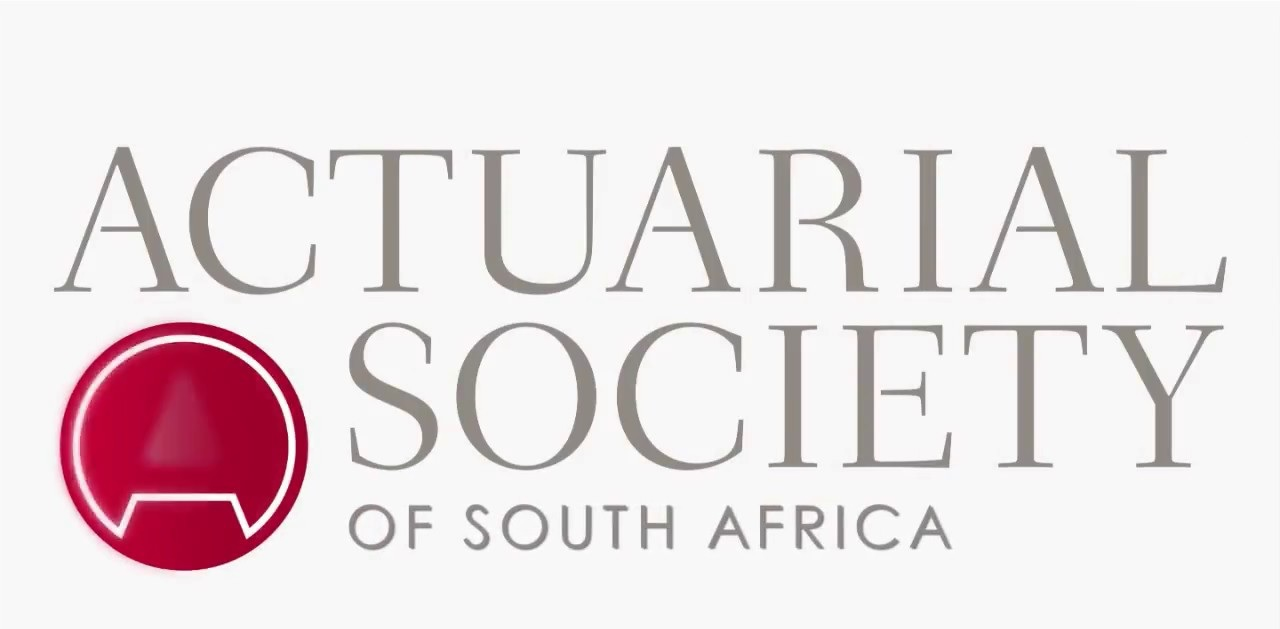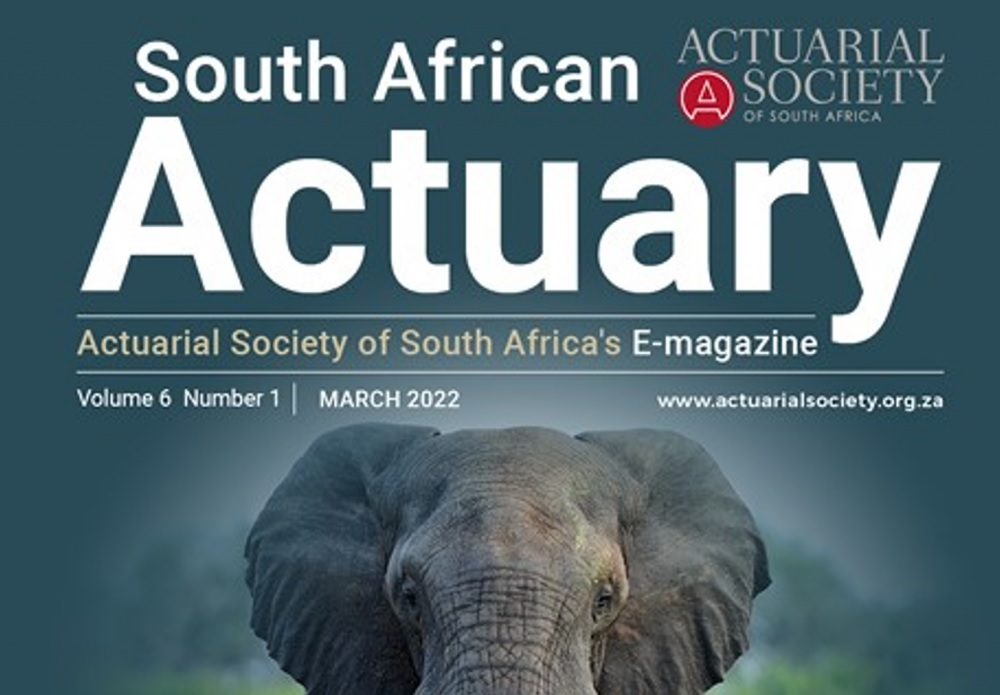1. Home
2. »
3. Syllabus
4. »
5. Actuarial Society Foundation Technical Statis...
All posts from

# Actuarial Society Foundation Technical Statistics Subject A111 Syllabi 2022

Organisation : Actuarial Society
Course Name : Foundation Technical
Subject Name : Actuarial Statistics
Subject Code : Subject A111
Year : 2022
Website : https://www.actuarialsociety.org.za

Want to comment on this post?

## Actuarial Society Statistics Syllabus

Actuarial Society Foundation Technical Actuarial Statistics Subject A111 Syllabus / Syllabi 2022.

Related / Similar Syllabi : Actuarial Society Foundation Technical Business Economics Subject A112 Syllabi 2022## Actuarial Society Statistics Objectives Syllabus

1. Random variables and distributions (20%):
1.1 Define basic univariate distributions and use them to calculate probabilities, quantiles and moments.
1.1.1 Define and explain the key characteristics of the discrete distributions: geometric, binomial, negative binomial, hypergeometric, Poisson and uniform on a finite set.
1.1.2 Define and explain the key characteristics of the continuous distributions: normal, lognormal, exponential, gamma, chi-square, 𝑡, 𝐹, beta and uniform on an interval.
1.1.3 Evaluate probabilities and quantiles associated with distributions (by calculation or using statistical software as appropriate).
1.1.4 Define and explain the key characteristics of the Poisson process and explain the connection between the Poisson process and the Poisson distribution.
1.1.5 Generate basic discrete and continuous random variables using the inverse transform method.
1.1.6 Generate discrete and continuous random variables using statistical software.

1.2 Independence, joint conditional distributions, linear combinations of random variables:
1.2.1 Explain what is meant by jointly distributed random variables, marginal distributions and conditional distributions.
1.2.2 Define the probability function/density function of a marginal distribution and of a conditional distribution.
1.2.3 Specify the conditions under which random variables are independent.
1.2.4 Define the expected value of a function of two jointly distributed random variables, the covariance and correlation coefficient between two variables, and calculate such quantities.
1.2.5 Define the probability function/density function of the sum of two independent random variables as the convolution of two functions.
1.2.6 Derive the mean and variance of linear combinations of random variables.
1.2.7 Use generating functions to establish the distribution of linear combinations of independent random variables.

1.3 Expectations, conditional expectations:
1.3.1 Define the conditional expectation of one random variable given the value of another random variable, and calculate such a quantity.
1.3.2 Show how the mean and variance of a random variable can be obtained from expected values of conditional expected values, and apply this.

1.4 Generating functions:
1.4.1 Define and determine the moment generating function of random variables.
1.4.2 Define and determine the cumulant generating function of random variables.
1.4.3 Use generating functions to determine the moments and cumulants of random variables, by expansion as a series or by differentiation, as appropriate.
1.4.4 Identify the applications for which a moment generating function, a cumulant generating function and cumulants are used, and the reasons why they are used.
1.5 Central Limit Theorem – statement and application
1.5.1 State the Central Limit Theorem for a sequence of independent, identically distributed random variables.
1.5.2 Generate simulated samples from a given distribution and compare the sampling distribution with the Normal.

2. Data Analysis (15%):
2.1 Data analysis:
2.1.1. Describe the possible aims of a data analysis (e.g. descriptive, inferential, and predictive).
2.1.2. Describe the stages of conducting a data analysis to solve real-world problems in a scientific manner and describe tools suitable for each stage.
2.1.3. Describe sources of data and explain the characteristics of different data sources, including extremely large data sets.
2.1.4. Explain the meaning and value of reproducible research and describe the elements required to ensure a data analysis is reproducible.

2.2 Exploratory data analysis:
2.2.1 Describe the purpose of exploratory data analysis.
2.2.2 Use appropriate tools to calculate suitable summary statistics and undertake exploratory data visualizations.
2.2.3 Define and calculate Pearson’s, Spearman’s and Kendall’s measures of correlation for bivariate data, explain their interpretation and perform statistical inference as appropriate.
2.2.4 Use Principal Components Analysis to reduce the dimensionality of a complex data set.

2.3 Random sampling and sampling distributions:
2.3.1 Explain what is meant by a sample, a population and statistical inference.
2.3.2 Define a random sample from a distribution of a random variable.
2.3.3 Explain what is meant by a statistic and its sampling distribution.
2.3.4 Determine the mean and variance of a sample mean and the mean of a sample variance in terms of the population mean, variance and sample size.
2.3.5 State and use the basic sampling distributions for the sample mean and the sample variance for random samples from a normal distribution.
2.3.6 State and use the distribution of the t-statistic for random samples from a normal distribution.
2.3.7 State and use the F distribution for the ratio of two sample variances from independent samples taken from normal distributions.

3 Statistical interference (20%):
3.1 Estimation and estimators:
3.1.1 Describe and apply the method of moments for constructing estimators of population parameters.
3.1.2 Describe and apply the method of maximum likelihood for constructing estimators of population parameters.
3.1.3 Define the terms: efficiency, bias, consistency and mean squared error.
3.1.4 Define and apply the property of unbiasedness of an estimator.
3.1.5 Define the mean square error of an estimator, and use it to compare estimators.
3.1.6 Describe and apply the asymptotic distribution of maximum likelihood estimators.
3.1.7 Use the bootstrap method to estimate properties of an estimator.

3.2 Confidence intervals:
3.2.1 Define in general terms a confidence interval for an unknown parameter of a distribution based on a random sample.
3.2.2 Derive a confidence interval for an unknown parameter using a given sampling distribution.
3.2.3 Calculate confidence intervals for the mean and the variance of a normal distribution.
3.2.4 Calculate confidence intervals for a binomial probability and a Poisson mean, including the use of the normal approximation in both cases.
3.2.5 Calculate confidence intervals for two-sample situations involving the normal distribution, and the binomial and Poisson distributions using the normal approximation.
3.2.6 Calculate confidence intervals for a difference between two means from paired data.
3.2.7 Use the bootstrap method to obtain confidence intervals